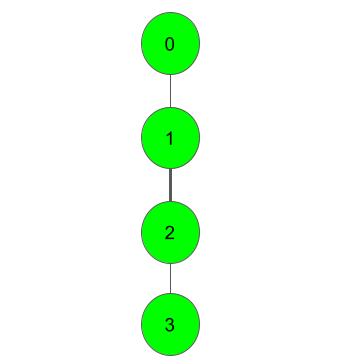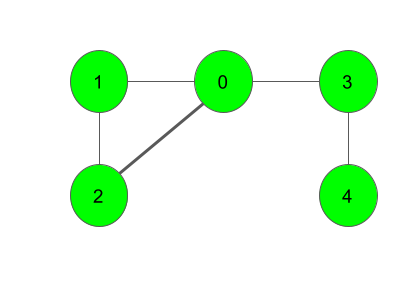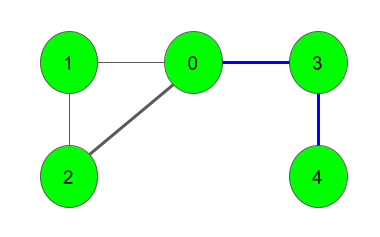Bridge edge in a graph
Medium Accuracy: 45.82% Submissions: 15254 Points: 4

Given a Graph of V vertices and E edges and another edge(c - d), the task is to find if the given edge is a Bridge. i.e., removing the edge disconnects the graph.

Example 1:

Input:c = 1, d = 2
Output:
1
Explanation:
From the graph, we can clearly see that
blocking the edge 1-2 will result in
disconnection of the graph. So, it is
a Bridge and thus the Output 1.



Example 2:

Input:c = 0, d = 2
Output:
0
Explanation:blocking the edge between nodes 0 and 2
won't affect the connectivity of the graph.
So, it's not a Bridge Edge. All the Bridge
Edges in the graph are marked with a blue
line in the above image.


You don't need to read input or print anything. Your task is to complete the function isBridge()
which takes number of vertices V, the number of edges E, an adjacency lsit adj and two integers c and d denoting the edge as input parameters and returns 1 if the given edge c-d is a Bridge. Else, it returns 0.

Expected Time Complexity: O(V + E).
Expected Auxiliary Space: O(V).

Constraints:
1
V,E 105
0
c, d V-1

We are replacing the old Disqus forum with the new Discussions section given below.

### Editorial

We strongly recommend solving this problem on your own before viewing its editorial. Do you still want to view the editorial?

#### My Submissions: